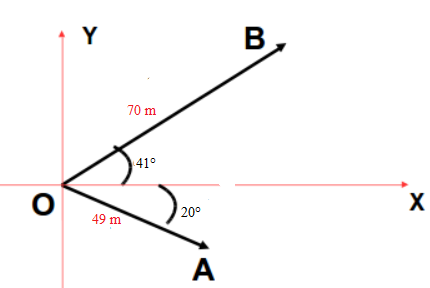# A vector A has a magnitude of 49.0 m and points in a direction 20.0 below the x axis. A second...

## Question:

A vector A has a magnitude of 49.0 m and points in a direction 20.0 below the x axis. A second vector, B, has a magnitude of 70.0 m and points in a direction 41.0 above the x axis. Find the magnitude and direction of the vector D.

## Resultant of Two Vectors :

If two vectors 'P' and 'Q' are inclined at angle {eq}\alpha {/eq} with each other, their resultant 'R' is given by

{eq}R^2=P^2+Q^2 +2PQ cos \alpha {/eq}

and the angle made by resultant with the direction of 'P' is given by

{eq}\tan \beta = \dfrac{ Q \sin \alpha }{P+Q \cos \alpha} {/eq}The magnitude of resultant vector 'D' is given by

{eq}D^2=49^2+70^2+2(49)(70)\cos(41+20) \\ \Rightarrow D=103\ m {/eq}

Direction from 49 m is given by

{eq}tan \alpha= \dfrac{70\sin61}{49+70\cos61} \\ \Rightarrow \alpha =36^\circ {/eq}